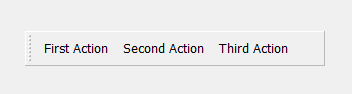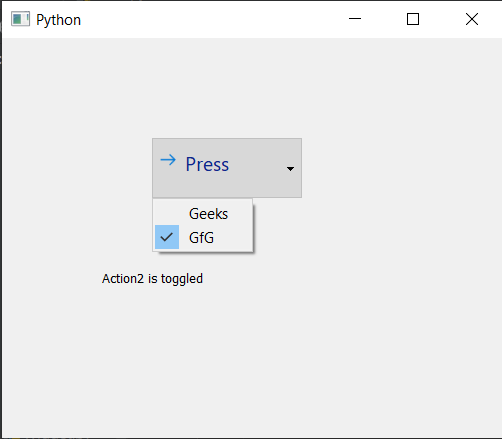Open in App
Not now

# PyQt5 – QAction

• Last Updated : 24 May, 2022

QAction : In PyQt5 applications many common commands can be invoked via menus, toolbar buttons, and keyboard shortcuts, since the user expects each command to be performed in the same way, regardless of the user interface used, QAction is useful to represent each command as an action. Actions can be added to menus and toolbars, and will automatically keep them in sync. For example, in a word processor, if the user presses a Bold toolbar button, the Bold menu item will automatically be checked. Below is how an action will look inside the tool barSyntax:

`action = QAction(name)`

This action can be added to toolbars or QMenus with the help of addAction and addActions method. Below are the some frequently used commands with the QAction

```setCheckable : To make QAction Checkable

setIcon : To add icon to the QAction

setText : To set the display name of the QAction

text : To get the display name of the QAction

setPriority : To set the priority of the action

triggered.connect : To connect an method with it when triggered signal is emitted```

Example : In this example we will create a main window having a tool bar, label and tool bar is consisting of QAction each having separate method connected to it, below is the implementation

## Python3

 `# importing libraries` `from` `PyQt5.QtWidgets ``import` `*` `from` `PyQt5 ``import` `QtCore, QtGui` `from` `PyQt5.QtGui ``import` `*` `from` `PyQt5.QtCore ``import` `*` `import` `sys`     `class` `Window(QMainWindow):`   `    ``def` `__init__(``self``):` `        ``super``().__init__()`   `        ``# setting title` `        ``self``.setWindowTitle("Python ")`   `        ``# setting geometry` `        ``self``.setGeometry(``100``, ``100``, ``500``, ``400``)`   `        ``# calling method` `        ``self``.UiComponents()`   `        ``# showing all the widgets` `        ``self``.show()`       `    ``# method for components` `    ``def` `UiComponents(``self``):`   `        ``# creating a tool bar` `        ``toolbar ``=` `QToolBar(``self``)`   `        ``# setting geometry to the tool bar` `        ``toolbar.setGeometry(``50``, ``100``, ``300``, ``35``)`     `        ``# creating QAction Instances` `        ``action1 ``=` `QAction("First Action", ``self``)` `        ``action2 ``=` `QAction("Second Action", ``self``)` `        ``action3 ``=` `QAction("Third Action", ``self``)`   `        ``# adding these actions to the tool bar` `        ``toolbar.addAction(action1)` `        ``toolbar.addAction(action2)` `        ``toolbar.addAction(action3)`   `        ``# creating a label` `        ``label ``=` `QLabel("GeeksforGeeks", ``self``)`   `        ``# setting geometry to the label` `        ``label.setGeometry(``100``, ``150``, ``200``, ``50``)`   `        ``# adding triggered action to the first action` `        ``action1.triggered.connect(``lambda``: label.setText("First Action Triggered"))`   `        ``# adding triggered action to the second action` `        ``action2.triggered.connect(``lambda``: label.setText("Second Action Triggered"))`   `        ``# adding triggered action to the third action` `        ``action3.triggered.connect(``lambda``: label.setText("Third Action Triggered"))`           `# create pyqt5 app` `App ``=` `QApplication(sys.argv)`   `# create the instance of our Window` `window ``=` `Window()`   `# start the app` `sys.exit(App.``exec``())`

Output :

Another example In this example we will create a commandlink button and add menu to it which is having QAction, below is the implementation

## Python3

 `# importing libraries` `from` `PyQt5.QtWidgets ``import` `*` `from` `PyQt5 ``import` `QtCore, QtGui` `from` `PyQt5.QtGui ``import` `*` `from` `PyQt5.QtCore ``import` `*` `import` `sys`     `class` `Window(QMainWindow):`   `    ``def` `__init__(``self``):` `        ``super``().__init__()`   `        ``# setting title ` `        ``self``.setWindowTitle("Python ")`   `        ``# setting geometry ` `        ``self``.setGeometry(``100``, ``100``, ``500``, ``400``)`   `        ``# calling method ` `        ``self``.UiComponents()`   `        ``# showing all the widgets ` `        ``self``.show()`   `        ``# method for components`   `    ``def` `UiComponents(``self``):` `        ``# creating a command link button` `        ``cl_button ``=` `QCommandLinkButton("Press", ``self``)`   `        ``# setting geometry ` `        ``cl_button.setGeometry(``150``, ``100``, ``150``, ``60``)`   `        ``# QActions ` `        ``action1 ``=` `QAction("Geeks", ``self``)` `        ``action2 ``=` `QAction("GfG", ``self``)`   `        ``# making action2 checkable` `        ``action2.setCheckable(``True``)`       `        ``# QMenu ` `        ``menu ``=` `QMenu()`   `        ``# adding actions to menu ` `        ``menu.addActions([action1, action2])`   `        ``# setting menu to the button ` `        ``cl_button.setMenu(menu)`     `        ``# creating label ` `        ``label ``=` `QLabel("GeeksforGeeks", ``self``)`   `        ``# setting label geometry ` `        ``label.setGeometry(``100``, ``200``, ``300``, ``80``)`   `        ``# making label multiline ` `        ``label.setWordWrap(``True``)`   `        ``# adding method to the action` `        ``action1.triggered.connect(``lambda``: label.setText("Action1 ``is` `triggered"))`   `        ``# adding method to the action2 when it get checked` `        ``action2.toggled.connect(``lambda``: label.setText("Action2 ``is` `toggled"))`       `# create pyqt5 app` `App ``=` `QApplication(sys.argv)`   `# create the instance of our Window ` `window ``=` `Window()`   `# start the app ` `sys.exit(App.``exec``()) `

Output :My Personal Notes arrow_drop_up
Related Articles